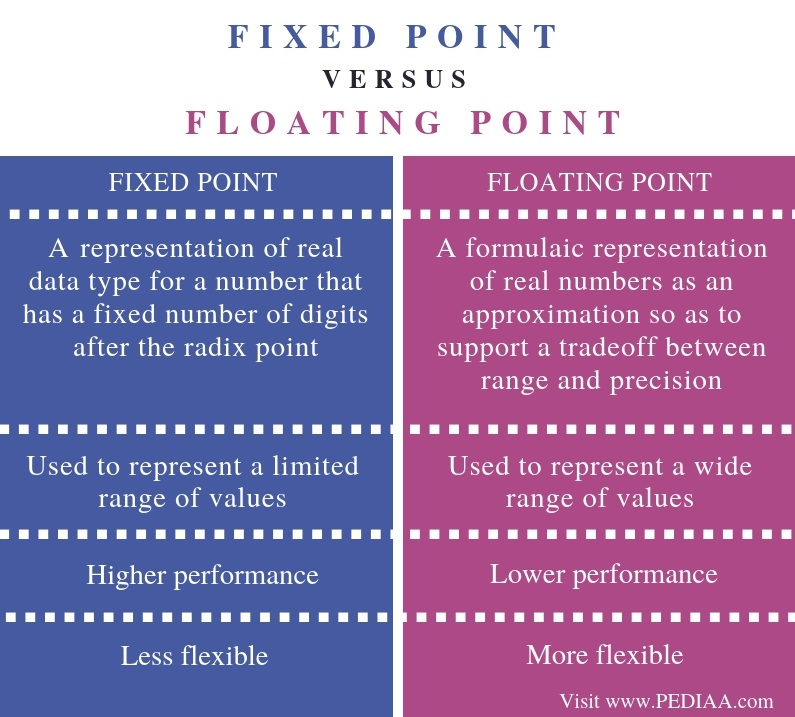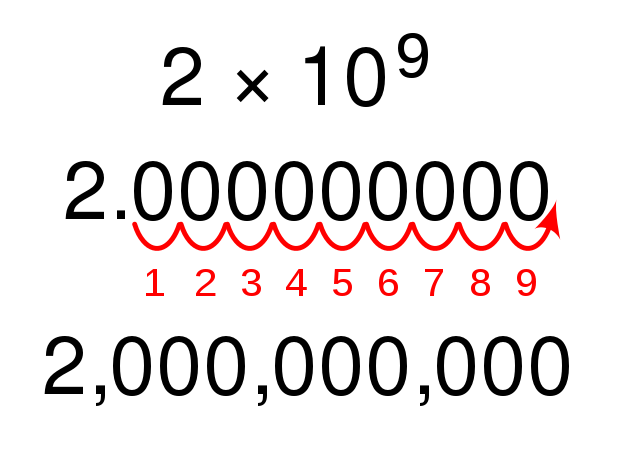Difference Between Fixed Point and Floating Point

The main difference between fixed point and floating point is that the fixed point has a specific number of digits reserved for the integer part and fractional part while the floating point does not have a specific number of digits reserved for the integer part and fractional part.

Fixed point and floating point are two ways of representing numbers. In fixed point, there is a specific number of digits to represent the integer section and fraction section. In other words, there is a fixed number of digits for each portion even though the number is very large or small. On the other hand, in floating point, there is no specific number of digits to represent integer section and fraction section. Floating point representation can cover a large range or numbers when compared to fixed point.

Key Areas Covered

1. What is a Fixed Point
– Definition, Functionality
2. What is a Floating Point
– Definition, Functionality
3. Difference Between Fixed Point and Floating Point
– Comparison of Key Differences

Key Terms

Fixed Point, Floating PointWhat is a Fixed Point

There are three sections in fixed point representation. They are the singed field, integer field, and the fractional field. Assume a number such as 1000.100. The 1 in the leftmost end is the signed field. It signifies whether the number is negative or positive. After that, the 000 is the integer field. The ‘.’ is the radix or decimal point. The number after the radix point is the fractional field.

In fixed point representation, the number of digits before and after the radix cannot be changed. Assume a number like + 20.05. Considering two digits in front of the radix and two digits after the radix, the minimum number that can be represented is -99.99 and the maximum number is +99.99. In this scenario, a number such as 20.223 cannot be represented as it has three digits after the radix point. As an alternative, the number can be represented as 20.22. This is called precision reduction. It is not the actual value, just an approximation.

Overall, fixed point representation allows improving the performance. On the other hand, it can only be used to represent a limited range of values.

What is Floating Point

Floating point representation can be used to overcome the limitations of fixed point representation. Therefore, most modern computers use floating point representation to store fractional numbers in memory. It can represent very large and very small numbers precisely. It is based on the scientific notation.Figure 2: Scientific Notation

A number in floating point representation is as follows.

+/- Mantissa x 10 exponent

The sign indicates whether the number is negative or positive. The mantissa is the significand or the fraction. 10 defines the base of the decimal.

For example, 22.33 can be represented as 2.233 x 101, 0.2233 x 102, 0.02233 x 103, etc. They all represent the same number. Floating point representation is not always unique.

Similarly, floating point representation can be applied to binary numbers. The formula is as follows. The base is 2.

+/- Mantissa x 2 exponent

Difference Between Fixed Point and Floating Point

Definition

Fixed point is a representation of real data type for a number that has a fixed number of digits after the radix point. Floating point is a formulaic representation of real numbers as an approximation so as to support a tradeoff between range and precision.

Number Representation

While fixed point can be used to represent a limited range of values, floating point can be used to represent a wide range of values.

Performance

The performance of the fixed point is higher than floating point.

Flexibility

Floating point representation is more flexible than fixed point representation.

Conclusion

Fixed point and floating point are two methods of representing numbers. The difference between fixed point and floating point is that fixed point has a specific number of digits reserved for the integer part and fractional part while floating point does not have a specific number of digits reserved for the integer part and fractional part.

Reference:

1. Floating Point Representation – Fixed Point, GATEBOOK Video Lectures, 24 July 2017, Available here.
2. Floating Point Representation | IEEE 754 Single Precision |, Education 4u, 21 Feb. 2018, Available here.

Image Courtesy:

1. “Scientific Notation Big” By Brian Brondel at English Wikibooks (CC BY-SA 2.5) via Commons Wikimedia